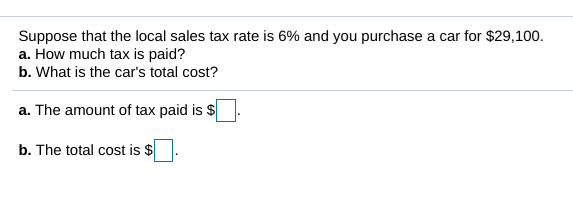# Suppose that the local sales tax rate is 6% and you purchase a car for \$29,100.a. How much tax is paid?b. What is the car's total cost?a. The amount of tax paid is \$b. The total cost is

Question
19 viewshelp_outlineImage TranscriptioncloseSuppose that the local sales tax rate is 6% and you purchase a car for \$29,100. a. How much tax is paid? b. What is the car's total cost? a. The amount of tax paid is \$ b. The total cost is fullscreen
check_circle

Step 1

Given

The sales tax is 6% of the price of the car.

The price of the car is \$29,100.

Step 2

Calculation:

a)

Compute the 6% of 29,100 as follows.

Step 3

b)

The total cost of the car is computed as follows.

The total cost of the car includes the amoun...

### Want to see the full answer?

See Solution

#### Want to see this answer and more?

Solutions are written by subject experts who are available 24/7. Questions are typically answered within 1 hour.*

See Solution
*Response times may vary by subject and question.
Tagged in

### Other